In :
%pylab inline
from IPython.display import display, Math, Latex
maths = lambda s: display(Math(s))
latex = lambda s: display(Latex(s))

Welcome to pylab, a matplotlib-based Python environment [backend: module://IPython.zmq.pylab.backend_inline].


A binary variable is good for representing something like a coin toss, but normally we want to have more states, such as the number rolled on a die. One way of representing such a variable is 1-of-K representation, where we have a vector $\mathbf{x}$ of size $K$ where state $k$ is represented by the $k^{th}$ element being 1 and all other elements being 0.

In :
def one_of(K, k):
# If k is an array, have each output row
# represent an element of k
k = array(k).flatten()
x = zeros((k.shape,K))
x[range(len(k)),k] = 1
return x


For example we can create a die

In :
class Die(object):
def __init__(self, mu=None, sides=6):
if mu == None:
mu =  * sides
# Normalise to be prob. dist.
self.mu = array(mu, float)/sum(mu)
self.sides = sides

def roll(self, n):
# sample using uniform rand num to index y axis of cdf
rolls = argmax(cumsum(self.mu)[:,newaxis] > random.random(n),0)
return one_of(self.sides, rolls)


And roll some numbers on a fair die

In :
fairdie = Die()
fairrolls = fairdie.roll(5)

print fairrolls
print "As (0-based) numbers:", argmax(fairrolls,axis=1)

[[ 0.  1.  0.  0.  0.  0.]
[ 0.  1.  0.  0.  0.  0.]
[ 0.  0.  0.  0.  0.  1.]
[ 1.  0.  0.  0.  0.  0.]
[ 0.  0.  1.  0.  0.  0.]]
As (0-based) numbers: [1 1 5 0 2]


If we have a loaded die, we can define a vector $\boldsymbol{\mu}$ of the probability for rolling each number

In :
# Say rolling a 1 is twice as likely as the other numbers
nums = [2, 1, 1, 1, 1, 1]
# normalise
mu = array(nums, float)/sum(nums)
print mu
latex(r"Probabilities, so normalised s.t. $\sum_{k=1}^{K} \mu_k = %s$" % round(sum(mu)))

[ 0.28571429  0.14285714  0.14285714  0.14285714  0.14285714  0.14285714]

Probabilities, so normalised s.t. $\sum_{k=1}^{K} \mu_k = 1.0$
In :
# roll some numbers on our loaded die


Out:
(array([284, 148, 146, 139, 135, 148]),
array([0, 1, 2, 3, 4, 5, 6]),
<a list of 6 Patch objects>)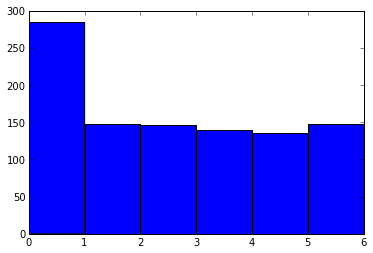Our probability distribution is just given by $\mu_k$ for each $k$, i.e. $p(k|\boldsymbol{\mu}) = \mu_k$ (a generalisation of the Bernoulli distribution, called the Categorical distribution)

But we want to use one-of-K to represent $k$ by vector $\mathbf{x}$, so we can write it as

$p(\mathbf{x}|\boldsymbol{\mu}) = \prod_{k=1}^K \mu_k^{x_k}$

This works because $\mu_k^{x_k}$ will be 1 for every element except that where $x_n$ = 1, so the product will give the $\mu_k$ for the state represented by $\mathbf{x}$. It is just a clever way of "picking out" the correct $\mu_k$.

In :
print mu**[0,1,0,0,0,0]

# only kth element is non-1

[ 1.          0.14285714  1.          1.          1.          1.        ]

In :
# Define our probability mass function
Die.pmf = lambda self,x: prod(self.mu**x, axis=-1)


Some examples with our weighted die

In :
x1 = [1,0,0,0,0,0] # "roll a one"
x2 = [0,1,0,0,0,0] # "roll a two"

maths(r"p(\mathbf{x}=%s|\boldsymbol{\mu}) = %s" % (x1,p1))
maths(r"p(\mathbf{x}=%s|\boldsymbol{\mu}) = %s" % (x2,p2))

$$p(\mathbf{x}=[1, 0, 0, 0, 0, 0]|\boldsymbol{\mu}) = 0.285714285714$$
$$p(\mathbf{x}=[0, 1, 0, 0, 0, 0]|\boldsymbol{\mu}) = 0.142857142857$$

Now consider the likelihood over N observations -- we simply need to multiply the previous result over all the samples

$p(D|\mu) = \prod_{n=1}^{N}p(\boldsymbol{x}_n|\boldsymbol{\mu})=$

$= \prod_{n=1}^{N}\prod_{k=1}^K \mu_k^{x_k}$

In :
Die.likelihood = lambda self, data: product(self.pmf(data))
Die.loglikelihood = lambda self, data: sum(log(self.pmf(data)))


Now given some observed dice rolls, we can plot how likely it is that the number one is weighted a certain way

In :
def plot_likelihood_1(r, *a, **kw):
prob_roll_1 = linspace(0,1,100)
ps = []

for p1 in prob_roll_1:
# Vary the probability of rolling a 1, and
# divide the remaining probability equally
# among the other 5 numbers
mus = (ones(6) * (1-p1))/5.
mus = p1
ps.append(Die(mus).likelihood(r))

plot(prob_roll_1, ps, *a, **kw)

# Plot some arbitrary data (where "0" means rolling a one)
plot_likelihood_1(one_of(6,[0,2,1,5,3,1,1]), 'red')
plot_likelihood_1(one_of(6,[1,0,1,2,0,1,0,0,0,0]), 'blue')

xlabel(r"$\mu_1$", size=22)

Out:
<matplotlib.text.Text at 0x3c45650>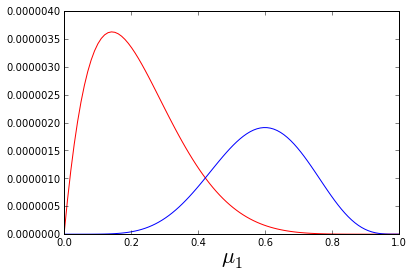In :
# Using some samples from our loaded die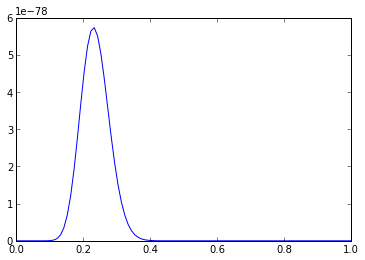We can also write the likelihood as

$p(D|\boldsymbol{\mu}) = \prod_{k=1}^K \mu_k^{m_k}$

Where $m_k$ is the number of data points where $x_k = 1$

Now to find the maximum likelihood solution for $\boldsymbol{\mu}$, we maximise $\log p(D|\boldsymbol{\mu})$ with the constraint that $\sum_{k=1}^{K}\mu_k = 1$, which we can enforce using a Lagrange multiplier $\lambda$.

$\frac{\partial}{\partial \mu_k}[\sum_{j=1}^{K}m_j \log \mu_j + \lambda(\sum_{j=1}^{K}\mu_j - 1)] = 0$

$\frac{m_k}{\mu_k} + \lambda(1 - 1) = 0$ (The partial derivative of terms in the summations where $j \neq k$ vanish)

$\mu_k = -\frac{m_k}{\lambda}$

Sub into $\sum_{k=1}^{K}\mu_k = 1$

$-\sum_{k=1}^{K} m_k = \lambda$

so $\lambda = -N$ (because the summation over the number of data points in each state gives the total number of data points)

And $\mu_k^{ML} = \frac{m_k}{N}$ (which is just the fraction of the data points where $x_k=1$)

Now consider the distribution over the $m_k$s, which would give the number of ones, twos, threes etc. rolled, the same way the Binomial gave us the number of heads. It is called the Multinomial:

$\operatorname{Mult}(m_1,m_2,...,m_K|\boldsymbol{\mu},N) = \binom{N}{m_1 m_2 ... m_K} \prod_{k=1}^K \mu_k^{m_k}$

In :
from scipy.misc import factorial

def multinomialpmf(ms, mu):
# ms: the number of observations of each state
ms = array(ms, float)
# We normalise our weights mu, so the input can be unnormalised
mu = array(mu, float)/sum(mu)
# The number of observations can be inferred from ms
N = sum(ms)
norm = factorial(N)/prod(factorial(ms))
return norm * prod(mu**ms)


We cannot really plot the multinomial directly, because of the many dimensions of $\boldsymbol{m}$.

But we can show the distribution of the number of ones rolled (constraining the other numbers), and with various weighted dice.

In :
n = 15 # max number of ones
m1s = arange(n+1)
N = 35

for w1 in [1,2,3]:
probs = []
for m1 in m1s:
# constrain so always sums to N
ms = [m1,5,5,5,5,n-m1]
assert sum(ms) == N
probs.append(multinomialpmf(ms,[w1,1,1,1,1,1]))
plot(m1s, probs, 'o--', label='"one" of weight %s' % w1)

xlabel("$m_1$ (the number of ones rolled)", size=15)
legend(loc='upper left')
title("Probability of rolling $m_1$ ones")

Out:
<matplotlib.text.Text at 0x40e3d10>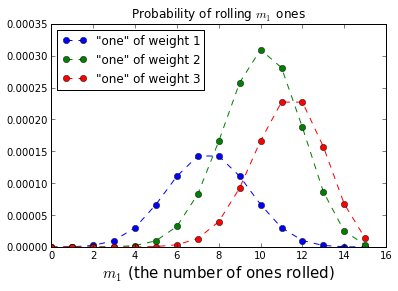And we can plot the distribution over a magical 3-sided die in 2D with a colourmap (only showing two variables on the axes, since the third can be inferred as they sum to N)

In :
N = 50
ms = arange(N+1)

# default to NaN (so white background for region where sum>N)
o = ones((N+1,N+1)) * NaN

for m1 in ms:
for m2 in arange((N-m1)+1):
m3 = N - m1 - m2
assert (m1 + m2 + m3) == N
assert (m3 >= 0)
o[m2,m1] = multinomialpmf([m1,m2,m3],[2,1,1])

imshow(o, origin='lower')
xlabel("number of ones")
ylabel("number of twos")
colorbar()
title('pmf of "three sided" die with weights [2,1,1]')

Out:
<matplotlib.text.Text at 0x42d3d10>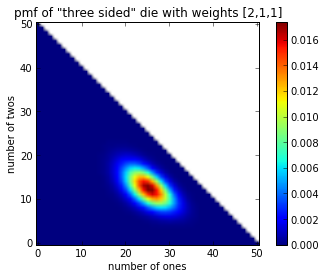In :


In :